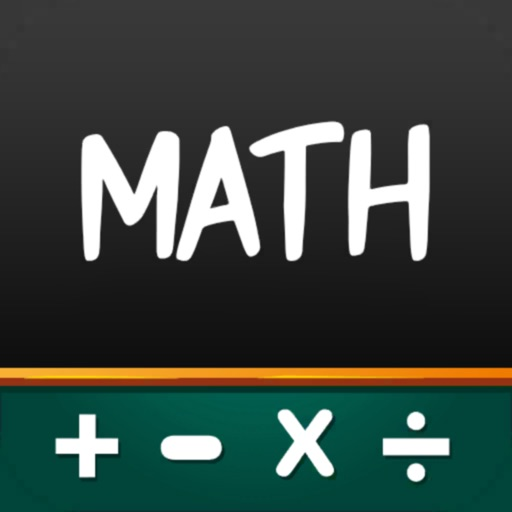If you are searching for kids math games then this is the best math game for your kids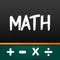Math Game - Brain IQ Puzzles

by Awais Naseer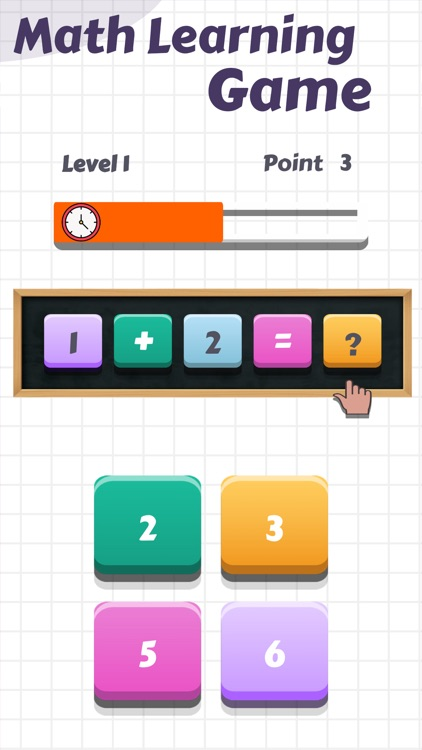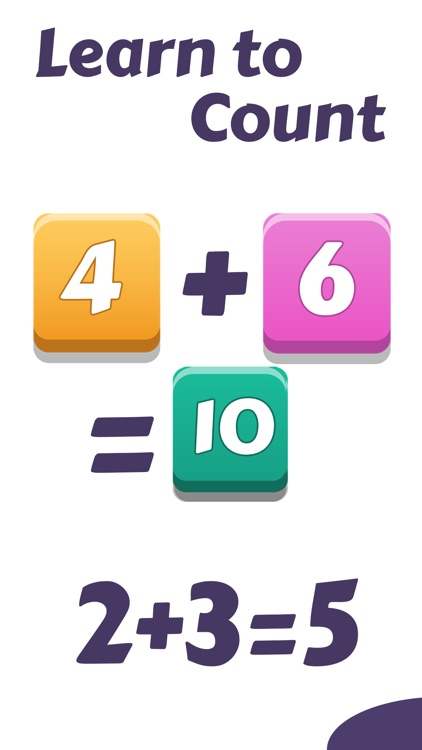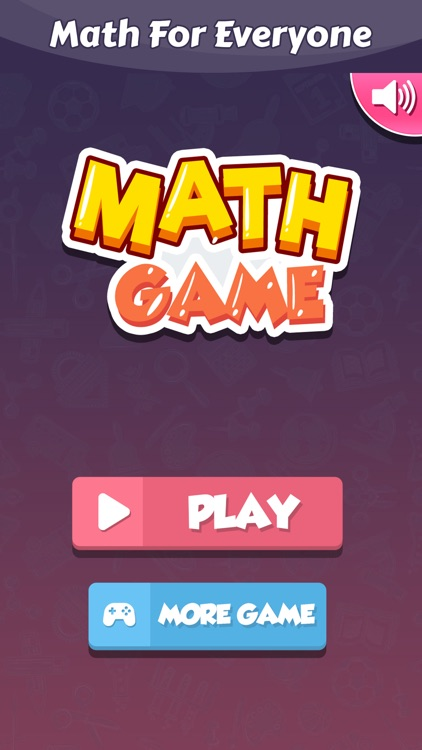If you are searching for kids math games then this is the best math game for your kids. Math Game is a free learning game that is made to teach your kid numbers and mathematics.App Details

Version
1.0
Rating
NA
Size
39Mb
Genre
Education Trivia Puzzle
Last updated
October 14, 2021
Release date
October 14, 2021

App Screenshots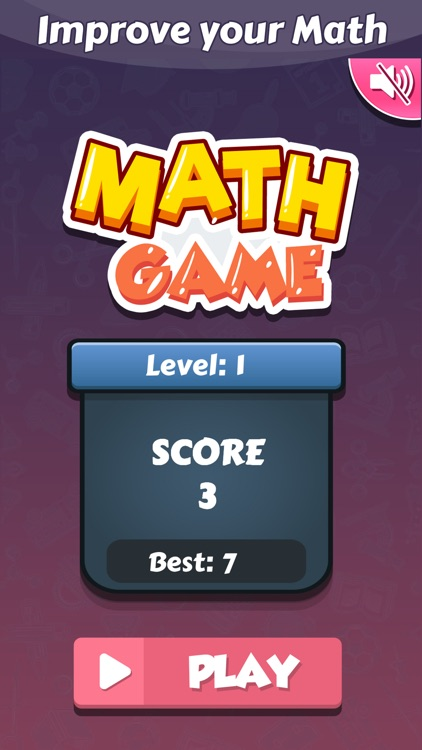App Store Description

If you are searching for kids math games then this is the best math game for your kids. Math Game is a free learning game that is made to teach your kid numbers and mathematics.
Math games increase the intelligence of kids and boost up their brains. You can solve the math riddle with addition, subtraction, multiplication, and division. When you solve a math riddle then you increase your points and win a level. Kids learn math riddles with arithmetic games. This Math game is completely free for adults and kids.
Math Game is a learning place for kids as well as adults who face problems in calculation. You can enhance your mathematical thinking by playing problem-solving games. Logic games improve your Iq level.
In this math game if you solve one math problem in a few seconds you can earn one point and when you earn points then you win one level.
This math kids app is offline and a free game. The purpose of making arithmetic games is that you can learn mathematics by playing this math problem solver. Math apps for kids enlarge mental skills, improve memory, focus, and mental speed. With arithmetic games, your brain will work quickly and more logically.
Math puzzles games keep your kid's brain Socratic and intelligent.
Math puzzle game is a superb introduction to counting, addition, and subtraction for all ages. This brain training game is very helpful to solve a math riddle fastest.

Key features of this math game:

Learn addition, subtraction, multiplication, and division in this math game.
Solve math riddles and earn levels.
Free math game for kids and adults.
Amazing math game, math puzzle, math riddle,iq game, arithmetic game, all in one.
Offline math kids apps.